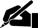# Term Paper: Microeconomics

Pages: 1 (351 words)  ·  Bibliography Sources: 0  ·  Topic: Business  ·  Buy This Paper

Microeconomics

The following is the complete elasticity chart. There were a couple of blanks where the denominator was zero that did not yield a usable result:

Price

Demand

Total Revenue

% Price

% Quant

Elasticity

#DIV/0!

#DIV/0!

Exercise

1a) If the initial price is \$400, then demand is 10000 - (400)(10) = 6000. If the price is \$500, then the demand is 10000 -- (500)(10) = 5000. The elasticity between these two prices is as follows:

% change in demand / % change in price

The change in demand is (4000-5000)/(5000) = -20%

The change in price is (500-400)/400 = 25%

The elasticity between \$400 and \$500 therefore is -20/25 = -0.8

The concept is elasticity reflects the degree to which the demand for a good is affected by the price of a good. To calculate elasticity, you need a change in price and a change in demand. When the price changes, elasticity tells us how much demand for that good is expected to change. In this case, every additional dollar is price will result… [END OF PREVIEW]

### Ordering Options:

1.  Download Full Paper (1 Pages)

or

2.  Let us write a NEW paper for you!Most popular!

#### Perfect Competition in Microeconomics Term Paper…

Cite This Term Paper:

APA Format

Microeconomics.  (2013, November 12).  Retrieved August 18, 2019, from https://www.essaytown.com/subjects/paper/microeconomics-following/2978470

MLA Format

"Microeconomics."  12 November 2013.  Web.  18 August 2019. <https://www.essaytown.com/subjects/paper/microeconomics-following/2978470>.

Chicago Format

"Microeconomics."  Essaytown.com.  November 12, 2013.  Accessed August 18, 2019.
https://www.essaytown.com/subjects/paper/microeconomics-following/2978470.